# SSC EXAMS | Reasoning Ability Practice Questions (Day-25)

Dear Aspirants, Here we have given the Important SSC Exam 2019 Practice Test Papers. Candidates those who are preparing for SSC 2019 can practice these questions to get more confidence to Crack SSC 2019 Examination.

[WpProQuiz 5195]

1) A cube is painted on red on all six sides. Then, it is cut into 1331 equal cubes. How many cubes are colored with only one side?

a) 978

b) 723

c) 482

d) 486

2)  ‘JIO’ is written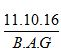as  in a fixed code language / pattern ‘PIO’ is written as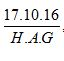then ‘TEA’ is written in the same code language / pattern.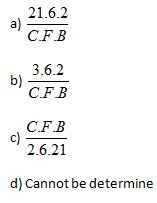3) Find the odd from the following given group?

a) 25 – Q

b) 21 – E

c) 22 – H

d) 32 – J

4) If ‘GEOMETRY’ is written as Y33 R25 T26 E10 M17 O18 E17 G8 , then ALGEBRA is written as-

a) A18, S24, B71, D6, G10, L14

b) A8, T24, B7, F6, G10, O14

c) A8, R24, C7, E6, G10, L4

d) A8 R24 B7 E9 G18 L14 A2

5) In the following question below are given some statements followed by some conclusions. Taking the given statements to be true even if they seem to be at variance from commonly known facts, read all the conclusions and then decide which of the given conclusion logically follows the given statements

Statement

I. M ≤ E, II. M ≥ D, III. D ≰ G

Conclusion:

I. E ≥ D

II. M > G

III. G ≰ E

a) Only I and II are true

b) Only II and III true

c) All I, II and III true

d) None is true.

6) Do the following series complete the following?

ab _ abc _ _ c ab _ _ bc

a) c a b c a

b) c a b a c

c) a c bc

d) a c b a

7) If a mirror is placed on the MN line, given answer – which of the shapes will be a question – the correct image of the shape?

Question figure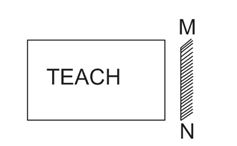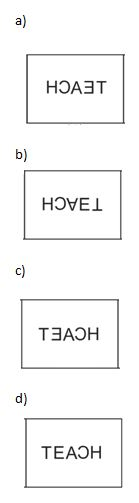8) If 25 ÷ 5 = 35 and 26 ÷ 13 = 32 So, Similarly 17 ÷ 32 = ?

a) 34

b) 51

c) 85

d) 40

9) If ‘X’ denotes ‘+’, ‘–‘denotes ‘’, ‘+’ denotes ‘÷’ and ‘÷’ denotes ‘–‘. Then find the value of- 10 × 20÷ 15 + 5 –

a) 25

b) 27

c) 3

d) 18

10)

In the following question below are given some statements followed by some conclusions. Taking the given statements to be true even if they seem to be at variance from commonly known facts, read all the conclusions and then decide which of the given conclusion logically follows the given statements

Statement:

I. All are mobile phones.

II. Any phone is not T.V.

Conclusion:

I. No Mobile is T.V

II. Some phones are mobile

a) Only I is true

b) Only II true

c) Both are true

d) None of these

We know that when all the surface of the cube is colored with the same color, only one surface of the central cube is colorful.

Number of Central Cubes = 6 (∛number of smaller cubes – 2)2

= 6 (11– 2)2 = 6×81= 486.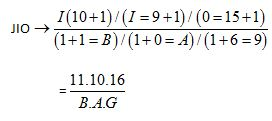And Similarly, PIO =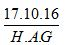So TEA →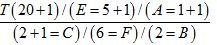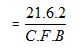25 ⇒ 2 × 5 + (2 + 5) = 17 → Q.

21 ⇒ 2 × 1 + (2 + 1) = 5 → E

22 ⇒ 2 × 2+ (2 + 2) = 8 ⇒ H

So, 32 ⇒ 3 × 2+ (3 + 2) = 11 ⇒ K

So, 32 – J (Here is odd-one).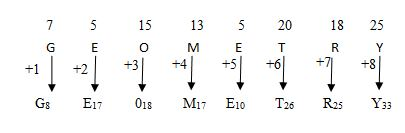Reverse Oder of this sequence Y33, R 25, T 26, E 10, M 17, O18, E17, G8

Similarly,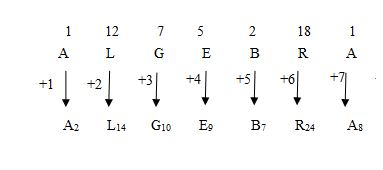Reverse order of the sequence => A8,  R24, B7, E6, G10, L14

G < D ≤ M ≤ E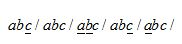25 ÷ 5 (2 + 5) × 5 = 35;

26 ÷ 13 (2 + 6) × (1 + 3) = 32;

17 ÷ 32 (1 + 7) × (3 + 2) = 40

10 × = 0 ÷15 + 5 – 1

= 10 + 20 – 15 ÷ 5 × 1

= 10 + 20 – 3

= 30 – 3 = 27.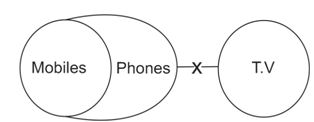According to the figure above.

Conclusion I and II are both true.